# Golang 数组的特点

• 固定长度：这意味着数组不可增长、不可缩减。想要扩展数组，只能创建新数组，将原数组的元素复制到新数组。
• 内存连续：这意味可以在缓存中保留的时间更长，搜索速度更快，是一种非常高效的数据结构，同时还意味着可以通过数值的方式(arr[index])索引数组中的元素。
• 固定类型：固定类型意味着限制了每个数组元素可以存放什么样的数据，以及每个元素可以存放多少字节的数据。

arr1 := int{10,20,30,40,50}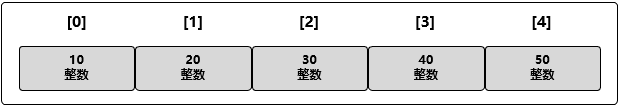# 数组的声明与初始化

var arr1 int

package main
import "fmt"
func main(){
var arr1 int
fmt.Println(arr1)     // 输出为：[0 0 0 0 0]
}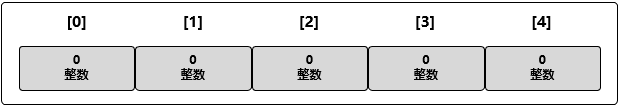arr2 := int{10,20,30,40,50}

arr3 := […]int{10,20,30,40,50}

//  用具体值初始化索引为 1 和 3 的元素
arr4 := int{1:20,3:40}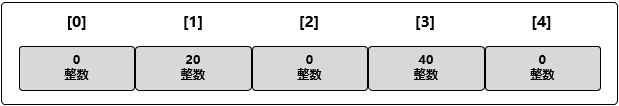# 访问与修改数组元素

package main
import "fmt"
func main(){
arr := int{10,20,30,40,50}
for i := 0; i < len(arr); i++ {
fmt.Printf("At index %d is %d\n", i, arr[i])
}
}

At index 0 is 10
At index 1 is 20
At index 2 is 30
At index 3 is 40
At index 4 is 50

for index,value := range arr {
fmt.Printf("At index %d is %d\n", index, value)
}

arr := int{10,20,30,40,50}
arr = 35

arr := *int{0: new(int), 1: new(int)}

new(TYPE) 函数会为一个 TYPE 类型的数据结构划分内存并执行默认的初始化操作，然后返回这个数据对象的指针，所以 new(int) 表示创建一个 int 类型的数据对象，同时返回指向这个对象的指针。

// 为索引为 0 和 1 的元素赋值
*arr = 10
*arr = 20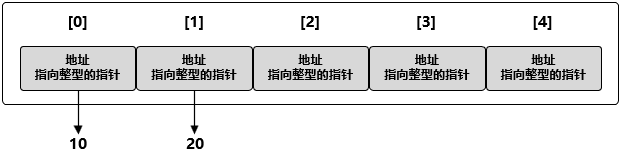arr = new(int)
arr = new(int)
arr = new(int)
*arr = 30

for i := 0; i < len(arr); i++ {
fmt.Printf("At index %d is %d\n", i, *arr[i])
}

At index 0 is 10
At index 1 is 20
At index 2 is 30
At index 3 is 0
At index 4 is 0

# 数组是值类型

var arr1 string
arr2 := string{"nick", "jack", "mark"}
// 把 arr2 的赋值(其实本质上是复制)到 arr1
arr1 = arr2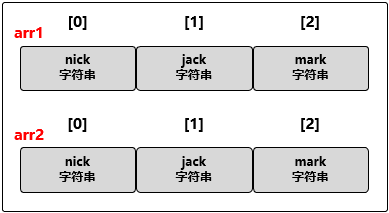// 声明第一个包含 4 个元素的字符串数组
var arr1 string
// 声明第二个包含 3 个元素的字符串数组，并初始化
arr2 := string{"nick", "jack", "mark"}
// 将 arr2 赋值给 arr1
arr1 = arr2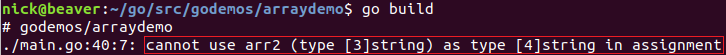// 声明第一个包含 4 个元素的字符串数组
var arr1 *string
// 声明第二个包含 3 个元素的字符串数组，并初始化
arr2 := *string{new(string), new(string), new(string)}
*arr2 = "nick"
*arr2 = "jack"
*arr2 = "mark"
// 将 arr2 赋值给 arr1
arr1 = arr2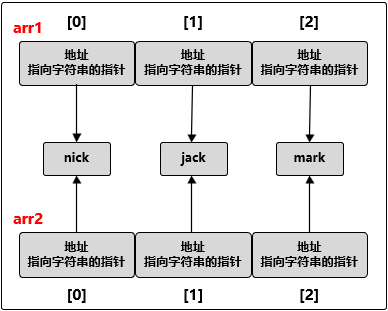# 把数组传递给函数

func showArray(array [1e6]int64){
// do something
}
var arr [1e6]int64
showArray(arr)

func showArray(array *[1e6]int64){
// do something
}
var arr [1e6]int64
showArray(&arr)

# 多维数组

Golang 的数组本身只有一个维度，但是我们可以组合多个数组从而创建出多维数组，下面是声明二维数组的实例代码：

// 声明一个二维整型数组，两个维度分别存储 4 个元素和 2 个元素
var arr int
// 使用数组字面量来声明并初始化一个二维整型数组
arr1 := int{{10, 11}, {20, 21}, {30, 31}, {40, 41}}
// 声明并初始化外层数组中索引为 1 和 3 的元素
arr2 := int{1: {20, 21}, 3: {40, 41}}
// 声明并初始化外层数组和内层数组的单个元素
arr3 := int{1: {0: 20}, 3: {1: 41}}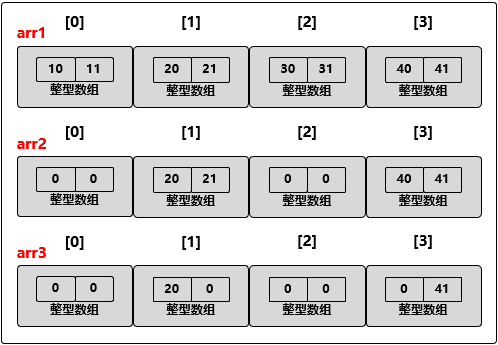arr1 = 666

// 将 arr1 的索引为 1 的维度复制到一个同类型的新数组里
var arr4 int = arr1
// 将外层数组的索引为 1、内层数组的索引为 0 的整型值复制到新的整型变量里
var value int = arr1

# 总结

Golang Array  types
《Go语言编程》
《Go语言实战》
go基础系列：数组

posted @ 2019-04-15 09:17 sparkdev 阅读(...) 评论(...) 编辑 收藏